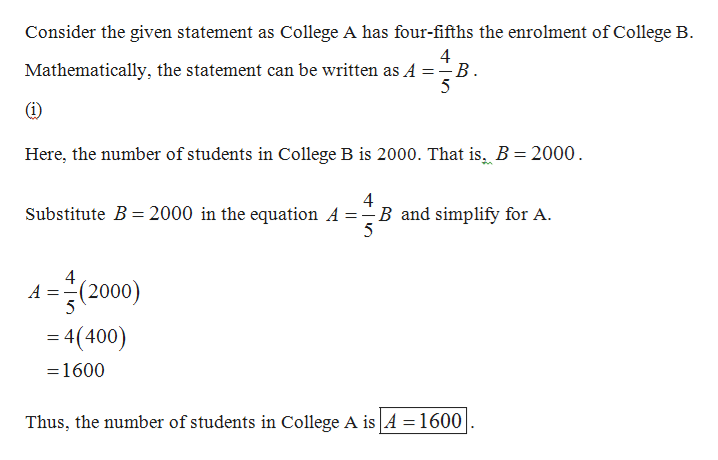# Suppose that College A has four-fifths the enrollment of College B.(i) If College B has 2000 students, how many students does College A have?(ii) If College A has 2000 students, how many students does College B have?

Question
14 views

Suppose that College A has four-fifths the enrollment of College B.

(i) If College B has 2000 students, how many students does College A have?

(ii) If College A has 2000 students, how many students does College B have?

check_circle

star
star
star
star
star
1 Rating
Step 1help_outlineImage TranscriptioncloseConsider the given statement as College A has four-fifths the enrolment of College B 4 Mathematically, the statement can be written as A =-B 5 Here, the number of students in College B is 2000. That is. B = 2000 4 B and simplify for A 5 Substitute B= 2000 in the equation A = (2000) A 5 4(400) 1600 Thus, the number of students in College A is A 1600 fullscreen

### Want to see the full answer?

See Solution

#### Want to see this answer and more?

Solutions are written by subject experts who are available 24/7. Questions are typically answered within 1 hour.*

See Solution
*Response times may vary by subject and question.
Tagged in

### Other